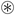## Wednesday, December 29, 2010

### Zipping with applicative functors in F#

In my last post I briefly described functors from a F# perspective. Now it's the turn of applicative functors. Since there were few to none concrete examples in my previous post, this time I'll start with an example. Hopefully everyone remembers Seq.zip. Remember also Seq.zip3 ? Just to recap, they combine sequences into sequences of pairs and triples respectively. The signatures are:

```Seq.zip : seq<'a> -> seq<'b> -> seq<'a * 'b>
Seq.zip3 : seq<'a> -> seq<'b> -> seq<'c> -> seq<'a * 'b * 'c>```

Now let's say we want zip5:

`Seq.zip5 : seq<'a> -> seq<'b> -> seq<'c> -> seq<'d> -> seq<'e> -> seq<'a * 'b * 'c * 'd * 'e>`

We could implement it with a nested zip3 and a flattening map:

```let zip5 a b c d e =
Seq.zip3 a b (Seq.zip3 c d e) |> Seq.map (fun (n,m,(o,p,q)) -> n,m,o,p,q)```

Or we could write it using an applicative functor, like this:

```let zip5 a b c d e =
puree (fun n m o p q -> n,m,o,p,q) <*> a <*> b <*> c <*> d <*> e```

I think you'll agree that the latter looks much cleaner, so let's see how we get there.

Applicative functors are defined by two operations: pure and `<*>` (actuallybut we can't write that in ASCII!). Again we can't express these generically in .NET's type system due to the lack of type constructor abstraction, but this is how their signatures would look like:

```pure : 'a -> 't<'a>
(<*>) : 't<'a -> 'b> -> 't<'a> -> 't<'b>```

I prefer the bracket-less ML way of expressing types, whenever possible:

```pure : 'a -> 'a 't
(<*>) : ('a -> 'b) 't -> 'a 't -> 'b 't```

Intuitively, pure lifts a value (usually a function) inside the domain of the applicative functor, and `<*>` applies the function inside the applicative to an applicative value, yielding another applicative value.

At this point, it might not be immediately clear that `<*>` is an application, but compare its signature with the regular function application operator (<|) and you'll see the similarity:

`(<|) : ('a -> 'b) -> 'a -> 'b`

Since pure is a reserved keyword in F# (it doesn't do anything yet, though) we'll use puree in our code instead.The applicative functor that enables the zip5 implementation above is known as ZipList:

```module ZipList =
let puree v = Seq.initInfinite (fun _ -> v)
let (<*>) f a = Seq.zip f a |> Seq.map (fun (k,v) -> k v)```

The signatures for ZipList:

```puree : 'a -> 'a seq
(<*>) : ('a -> 'b) seq -> 'a seq -> 'b seq```

In plain English, `<*>` in a ZipList applies a sequence of functions to a sequence of values, pairwise; while puree creates an infinite sequence of values (usually functions) ready to be applied to another sequence of values using `<*>`.

### Functors and applicatives

Since we're always using this ZipList in the form "`puree function <*> value`" we might as well wrap that in an operator:

`let (<!>) f a = puree f <*> a`

Sow now we can write zip5 a bit shorter:

```let zip5 a b c d e =
(fun n m o p q -> n,m,o,p,q) <!> a <*> b <*> c <*> d <*> e```

Let's take a moment to see the signature of `<!>`

`(<!>) : ('a -> 'b) -> 'a seq -> 'b seq`

This signature should look familiar to you. Yes, it's Seq.map. Applicative functors are also functors, which is quite obvious from its name but we hadn't seen it until now. So we can write a map (a.k.a. lift) function for every applicative functor exactly as the `<!>` operator above.

### Observing the law

Applicative functors, just as regular functors, need to satisfy some laws. Just as the last time, we'll use FsCheck, and again we'll have to cheat a little to get comparable types (we can't compare infinite sequences).

```open System.Linq
// limited comparison for infinite sequences
let toListFrom r a = Enumerable.Take(a, List.length r) |> Seq.toList

type ApplicativeLaws<'a,'b,'c when 'a:equality and 'b:equality>() =
static member identity (v: 'a list) =
let x = puree id <*> v
toListFrom v x = v
static member composition (u: ('a -> 'b) list) (v: ('c -> 'a) list) (w: 'c list) =
let toList = toListFrom u
let a = puree (<<) <*> u <*> v <*> w
let b = u <*> (v <*> w)
toList a = toList b
static member homomorphism (f: 'a -> 'b) x =
let toList a = Enumerable.Take(a, 10000) |> Seq.toList
let a = puree f <*> puree x
let b = puree (f x)
toList a = toList b
static member interchange (u: ('a -> 'b) list) (y: 'a) =
let toList = toListFrom u
let a = u <*> puree y
let b = puree ((|>) y) <*> u
toList a = toList b

FsCheck.Check.QuickAll<ApplicativeLaws<_,_,_>>()```

In the next post, we'll see how applicative functors relate to monads, with a parsing example.